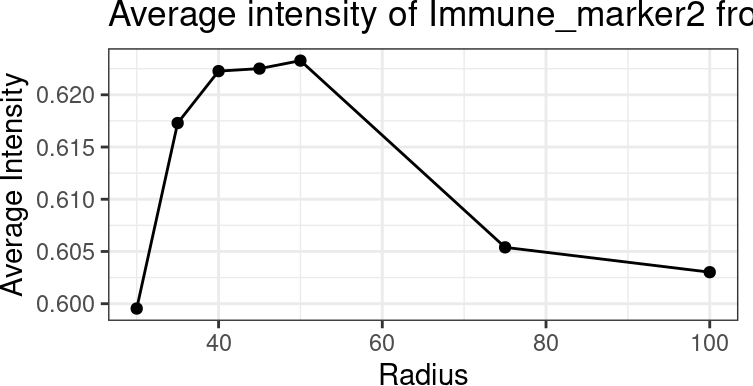``library(SPIAT)``

With SPIAT we can quantify cell colocalisation, which refers to how much two cell types are colocalising and thus potentially interacting.

In this vignette we will use an inForm data file that’s already been formatted for SPIAT with `format_image_to_spe()`, which we can load with `data()`. We will use `define_celltypes()` to define the cells with certain combinations of markers.

``````data("simulated_image")

# define cell types
formatted_image <- define_celltypes(
simulated_image,
categories = c("Tumour_marker","Immune_marker1,Immune_marker2",
"Immune_marker1,Immune_marker3",
"Immune_marker1,Immune_marker2,Immune_marker4", "OTHER"),
category_colname = "Phenotype",
names = c("Tumour", "Immune1", "Immune2", "Immune3", "Others"),
new_colname = "Cell.Type")``````

# 1 Cells In Neighbourhood (CIN)

We can calculate the average percentage of cells of one cell type (target) within a radius of another cell type (reference) using `average_percentage_of_cells_within_radius()`.

``````average_percentage_of_cells_within_radius(spe_object = formatted_image,
reference_celltype = "Immune1",
target_celltype = "Immune2",
``##  4.768123``

Alternatively, this analysis can also be performed based on marker intensities rather than cell types. Here, we use `average_marker_intensity_within_radius()` to calculate the average intensity of the target_marker within a radius from the cells positive for the reference marker. Note that it pools all cells with the target marker that are within the specific radius of any reference cell. Results represent the average intensities within a radius.

``````average_marker_intensity_within_radius(spe_object = formatted_image,
reference_marker ="Immune_marker3",
target_marker = "Immune_marker2",
``##  0.5995357``

To help identify suitable radii for `average_percentage_of_cells_within_radius()` and `average_marker_intensity_within_radius()` users can use `plot_average_intensity()`. This function calculates the average intensity of a target marker for a number of user-supplied radii values, and plots the intensity level at each specified radius as a line graph. The radius unit is microns.

``````plot_average_intensity(spe_object=formatted_image, reference_marker="Immune_marker3",
target_marker="Immune_marker2", radii=c(30, 35, 40, 45, 50, 75, 100))``````This plot shows low levels of Immune_marker3 were observed in cells near Immune_marker2+ cells and these levels increased at larger radii. This suggests Immune_marker2+ and Immune_marker3+ cells may not be closely interacting and are actually repelled.

# 2 Mixing Score (MS) and Normalised Mixing Score (NMS)

This score was originally defined as the number of immune-tumour interactions divided by the number of immune-immune interactions (Keren et al. 2018). SPIAT generalises this method for any user-defined pair of cell types. `mixing_score_summary()` returns the mixing score between a reference cell type and a target cell type. This mixing score is defined as the number of target-reference interactions/number of reference-reference interactions within a specified radius. The higher the score the greater the mixing of the two cell types. The normalised score is normalised for the number of target and reference cells in the image.

``````mixing_score_summary(spe_object = formatted_image, reference_celltype = "Immune1",
target_celltype = "Immune2", radius=100, feature_colname ="Cell.Type")``````
``````##   Reference  Target Number_of_reference_cells Number_of_target_cells
## 2   Immune1 Immune2                       338                    178
##   Reference_target_interaction Reference_reference_interaction Mixing_score
## 2                          583                             592    0.9847973
##   Normalised_mixing_score
## 2               0.9322379``````

# 3 Cross K function

Cross K function calculates the number of target cell types across a range of radii from a reference cell type, and compares the behaviour of the input image with an image of randomly distributed points using a Poisson point process. There are four patterns that can be distinguished from K-cross function, as illustrated in the plots below. (taken from here in April 2021).